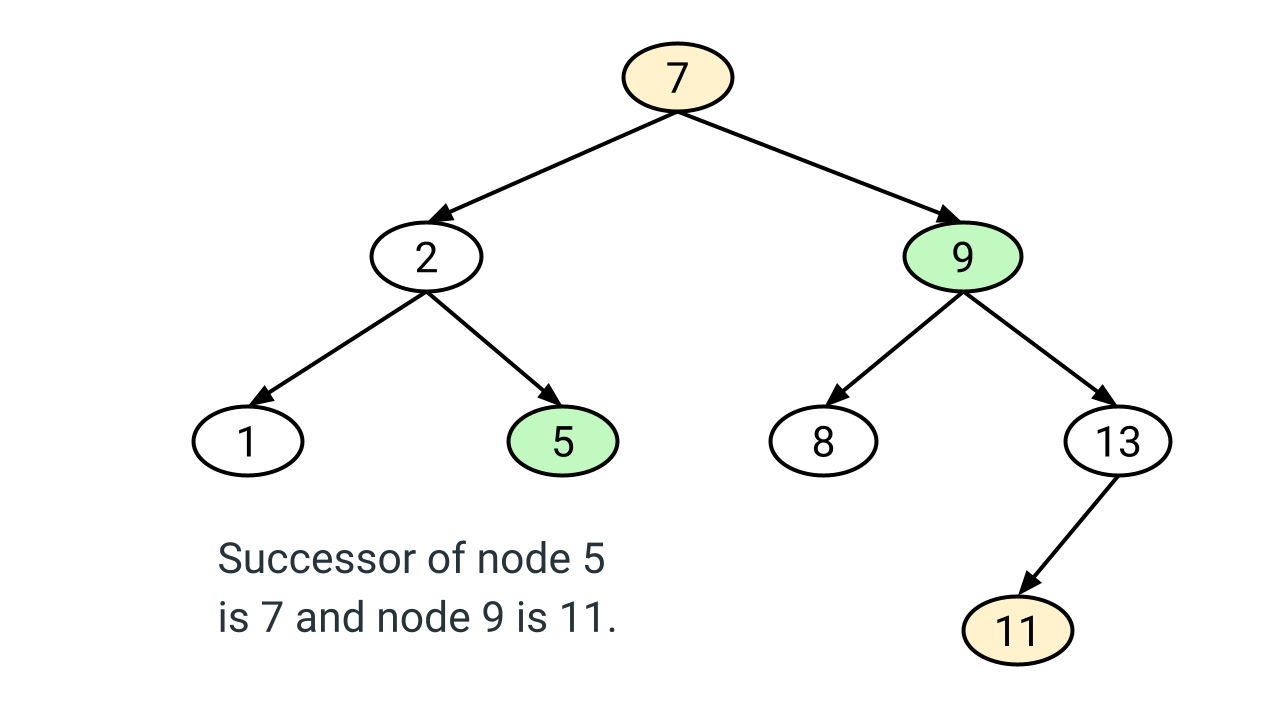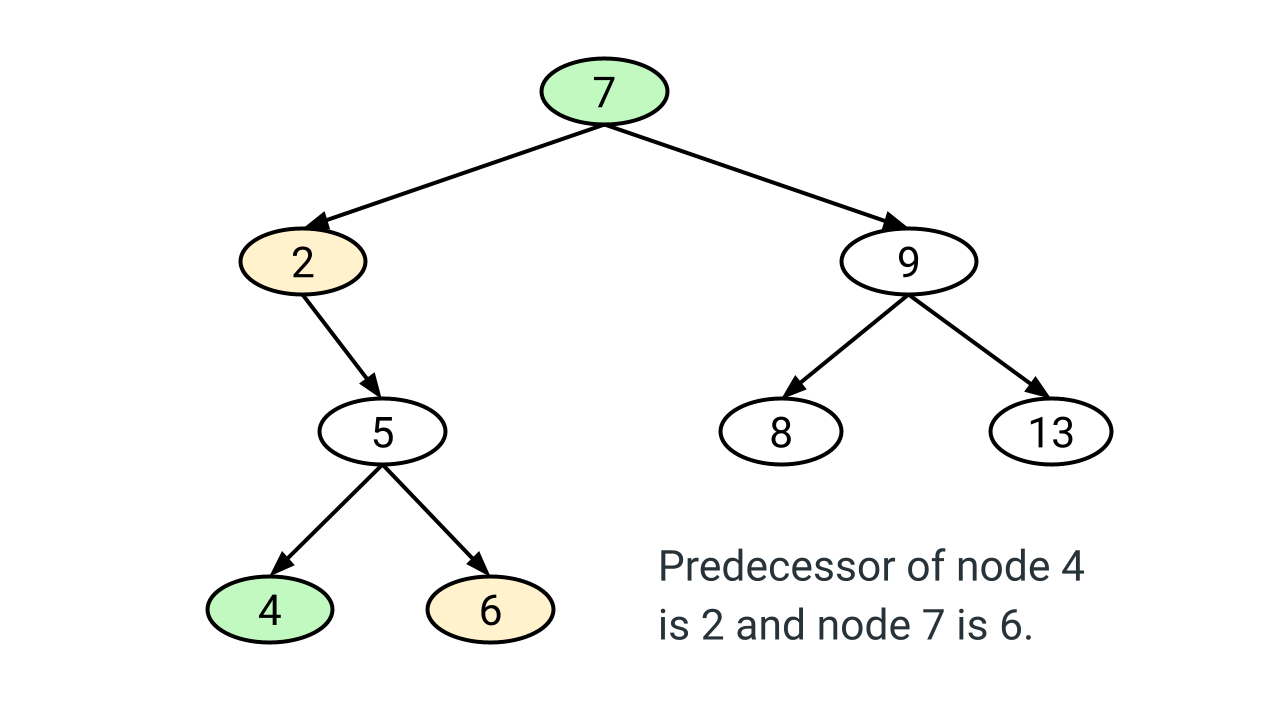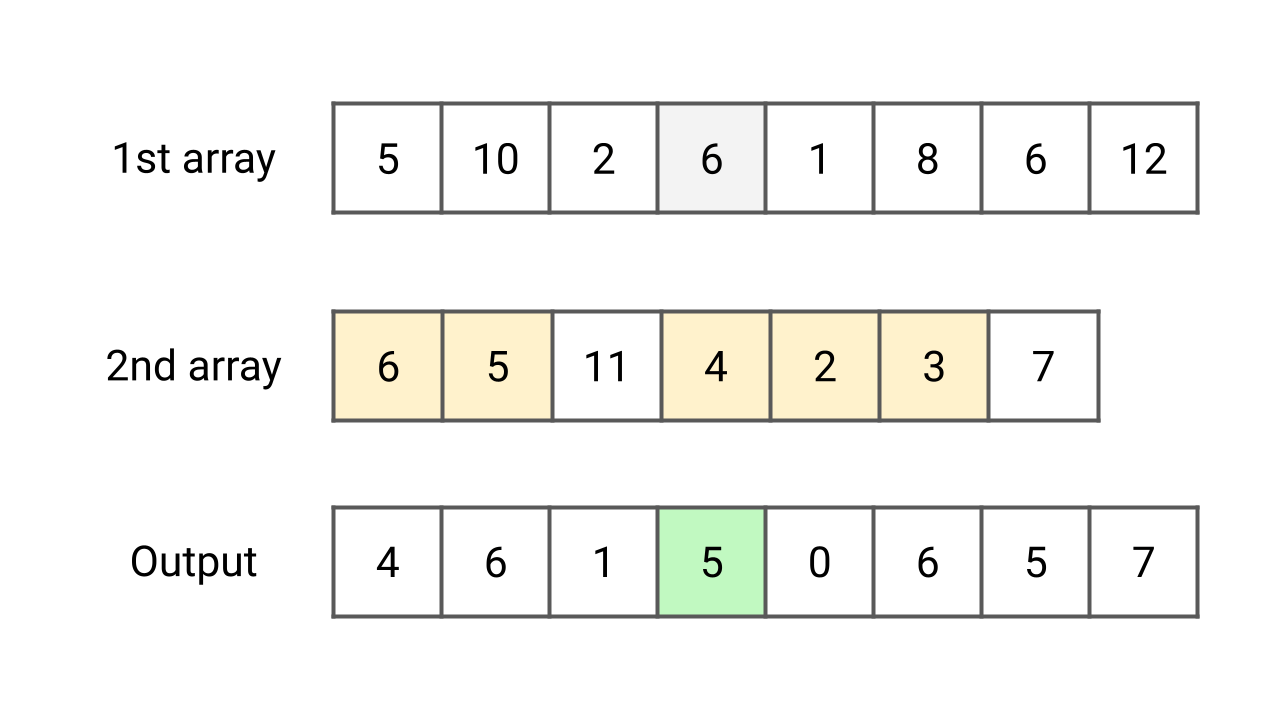# searchingPair sum in an array

Given an array of n integers and a target number, write a program to find whether a pair sum exists in the array or not. In other words, we need to check for a pair of elements in the array that sum exactly to the target value. Assume that all elements are distinct. Note: This is an excellent problem to learn problem solving using two pointers and hash table.Find Intersection of Two Arrays

Given two integer arrays, X[] and Y[] of size m and n, write a program to find intersection of these two arrays. The intersection is a list of common elements present in both arrays. Suppose m > n, all array elements are distinct and intersection elements can be in any order. Note: This is an excellent problem to learn problem solving using various approaches.Most Frequent Element in an Array

Given an array X[] of size n, write a program to find the most frequent element in the array, i.e. the element which occurs the most number of times. If multiple elements have maximum frequency, return the smallest (assume that at least one element is repeated). Note: This is an excellent problem to learn problem-solving using sorting and hash table.Inorder Successor of a Node in Binary Search Tree (BST)

Given a node x and the root of a binary search tree, write a program to find the in-order successor of node x. The in-order successor of node x is a node that will be visited just after node x during an in-order traversal. In other words, the in-order successor of a node x is the node with the smallest key greater than x->key.Inorder Predecessor of a Node in Binary Search Tree (BST)

Write a program to find the in-order predecessor of a given node x in a binary search tree (BST). An in-order predecessor of node x is the node that will be visited just before node x in the in-order traversal. If the node x is visited first (leftmost node in BST) then the predecessor of node x is NULL.Each value in 1st array count values less than or equal to in 2nd array

Given two unsorted arrays X[] and Y[] of size m and n. For each element in the first array X[] count the elements in the second array Y[] whose value is less than or equal to it. There can be duplicate elements in the arrays. Note: This is an excellent problem to learn problem solving using sorting and searching.Find Minimum and Maximum Element in an Array

Given an array X[] of size n, write a program to find the maximum and minimum elements while making the minimum number of comparisons. This is an excellent question to learn problem-solving using a single loop and divide and conquer approach. In the efficient single-loop solution, we increment the loop by two to optimize the comparison count.Binary Search Algorithm

Binary search is an efficient algorithm for searching a value in a sorted array using the divide and conquer idea. It compares the target value with the value at the mid-index and repeatedly reduces the search interval by half. The search continues until the value is found or the subarray size gets reduced to 0. The time complexity of the binary search is O(log n).Kth Smallest Element in an Array

Given an array and a positive integer k, write a program to find the kth smallest element in the array. This is an excellent problem to learn problem-solving using the max and min heap data structures. The quick-select approach (divide and conquer) is also worth exploring because it helps to optimize time complexity to O(n) on average.Row With Max 1s

Given a boolean 2D array, where each row is sorted. Find the row with the maximum number of 1s. This is an excellent matrix problem that can be solved in linear time complexity. The best part is — we are using the sorted order property and nested loops to improve the solution over the binary search approach.Find First and Last Position of Element in Sorted Array

Given an array of integers sorted in ascending order, the program should find the first and last occurrence of a given element. The goal is to design an algorithm with O(log n) time complexity. Note that this is an excellent question to learn problem-solving using binary search. Here, the binary search needs to be applied twice to solve this problem.N Repeated Element in Size 2N Array

In an array of size 2N, there are N + 1 unique elements, and exactly one of these elements is repeated n times. Write a program to return the N-repeated element in the given 2N size array. Note: This is an excellent problem to learn optimization using the mathematical approach. Sometimes mathematical insights into the problem can help us to get efficient solutions.Search in a row wise and column wise sorted matrix

You have given row-wise and column-wise sorted 2d matrix and integer k, write a program to search k in 2d matrix, i.e. find whether k is present or not. Each row is sorted from left to right, and the first integer of each row is greater than the last integer of the previous row. Note: This is an excellent problem to learn problem solving binary search in a 2d matrix.Find Maximum Element in a Bitonic Array

You are given an array of n integers that is first increasing and then decreasing. Write a program to find the maximum value in the array. Our goal should be to solve this problem using O(logn) time complexity. Note: This is an excellent problem to learn problem solving using binary search, where we modify binary search to get an efficient solution.Equilibrium Index of an Array

Write a program to find the equilibrium index of an array. An array's equilibrium index is an index such that the sum of elements at lower indexes equals the sum of elements at higher indexes. Note: This is an excellent coding question to learn time and space complexity optimization using a prefix array and a single loop using variables.Check if two arrays are equal or not

Given two integer arrays X[] and Y[], write a program to check if arrays are equal or not. Two arrays are equal if they have the same elements in any order. If there are repeated elements, then counts of repeated elements must also be the same for both arrays. Note: This is an excellent problem to learn problem solving using a hash table.AVL Tree

AVL Trees is a height-balanced BST named after its inventors, Adelson-Velsky and Landis. This is a variation of binary search trees, also known as self-balancing BST. The property of AVL Trees: The absolute difference of height between left and right subtree is at max 1, which could help us to perform bst operations in O(logn) time complexity.Hashing and Hash Table in Data Structure

Hashing is a technique to map (key, value) pairs into the hash table using a hash function. It uses an array of size proportional to the number of keys and calculates an array index from the key using a hash function. We use hashing/hash tables in several real life applications and solve various coding questions efficiently in data structures.Searching in Binary Search Tree (BST)

We start searching from root node and traverse a path downward in binary search tree. For each node in the path, compare target key k with the node key. If equal, search is successful. If k < node key, go to the left subtree. Similarly, if k > node key, go to the right subtree. In this blog, we have discussed recursive and iterative implementations of searching in BST.Introduction to Trie Data Structure

We mainly use trie data structure to process strings efficiently. It is a tree where each node represents a prefix or end of a word. The best thing is that the time complexity of trie operations depends on string length, which is independent of number of strings. Applications of trie: Autocomplete search, longest prefix matching, spell checker, etc.Hash Functions in Data Structure

We use hash functions to distribute keys in the hash table uniformly. In other words, a good hash function satisfies the assumption of uniform hashing, where each key is equally likely to hash to any slots in the hash table. This blog has discussed the design and properties of some popular hash functions used in algorithm and data structure.A* Search Algorithm

A* search algorithm is a popular technique for finding the shortest path in a graph from a given initial node to a destination node. For example, in video games, pathfinding can be used to move objects from their initial place to their destination via shortest route. So implementation of a star algorithm is popular in tile or map-based games.